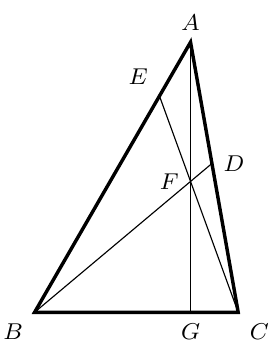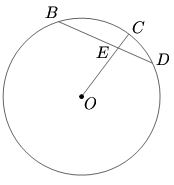#### Canada

###### back to index

Let $I$ be the incenter of $\triangle{ABC}$. $AI$, $BI$, and $CI$ intersect $\triangle{ABC}$'s circumcircle at $D$, $E$, and $F$, respectively. Show that $EF \perp AD$

Let $P(x) = x^n + a_{n-1}x^{n-1} + \cdots + a_1x + a_0$ be a polynomial with integral coefficients. Suppose that there exist four distinct integers $a, b, c, d$ with $P(a) = P(b) = P(c) = P(d) = 5$. Prove that there is no integer $k$ satisfying $P(k) = 8$.

In $\triangle{ABC}$, $\angle{BAC} = 40^\circ$ and $\angle{ABC} = 60^\circ$. Points $D$ and $E$ are on sides $AC$ and $AB$, respectively, such that $\angle{DBC}=40^\circ$ and $\angle{ECB}=70^\circ$. Let $F$ be the intersection point of $BD$ and $CE$. Show that $AF\perp BC$.$DEB$ is a chord of a circle such that $DE=3$ and $EB=5 .$ Let $O$ be the center of the circle. Join $OE$ and extend $OE$ to cut the circle at $C.$ Given $EC=1,$ find the radius of the circleFor pairwise distinct nonnegative reals $a,b,c$, prove that $$\frac{a^2}{(b-c)^2}+\frac{b^2}{(c-a)^2}+\frac{c^2}{(b-a)^2}>2$$

Equally divide each side of a triangle into $n$ parts and then connect these points to draw lines which are parallel to one of the triangle's sides. Find the number of parallelograms created by these lines.